Select Page

# Application of Derivatives CBSE Maths 12 Science MCQ Answers in English

Application of Derivatives CBSE Maths 12 Science MCQ Answers in English to enable students to get Answers in a narrative video format for the specific question.

Expert Teacher provides Application of Derivatives CBSE Maths 12 Science MCQ Answers through Video Answers in English language. This video solution will be useful for students to understand how to write an answer in exam in order to score more marks. This teacher uses a narrative style for a question from Application of Derivatives not only to explain the proper method of answering question, but deriving right answer too.

Please find the question below and view the Answer in a narrative video format.

Question:

## Similar Questions from CBSE, 12th Science, Maths, Application of Derivatives

Question 1 : A balloon, which always remains spherical has a variable radius. Find the rate at which its volume is increasing with the radius when the later is 10 cm.  (View Answer Video)

Question 2 : If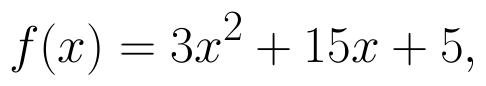then the approximate value of f(3.02) is : (View Answer Video)

Question 3 : Find the maximum profit that a company can make if the profit function is.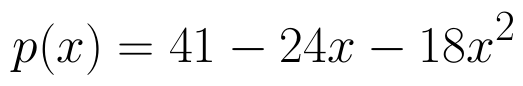(View Answer Video)

Question 4 : It is given that  at x=1, the function attains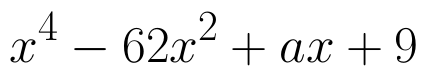its maximum value on the interval[0,2]. Find the value of a? (View Answer Video)

Question 5 : The length x of a rectangle is decreasing at the rate of 5cm/minute. and width y is increasing at the rate of 4cm/minute. When x=8cm and y=6 cm, find the rate of changes of:

### Continuity and Differentiability

Question 1 : If x and y are connected parametrically by the equation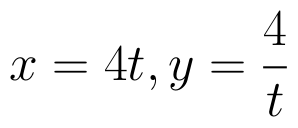, without eliminating the parameter, find. (View Answer Video)

Question 2 :  Find the second order derivative of the function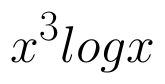. (View Answer Video)

Question 3 : If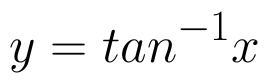, find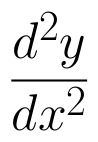in terms of y alone. (View Answer Video)

Question 4 : If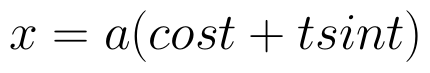and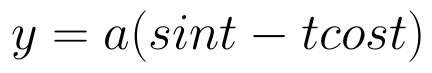, find. (View Answer Video)

Question 5 : Findfor the function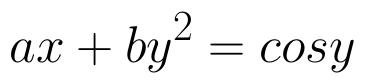. (View Answer Video)

### Linear Programming

Question 1 : The objective function is maximum or minimum, which lies on the boundary of the feasible region. (View Answer Video)

### Vector Algebra

Question 1 : Write the position vector of the point which divides the join of points with position vectors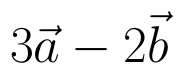and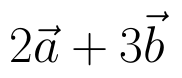in the ratio 2:1.  (View Answer Video)

Question 2 : If the vertices A, B, C of a triangle ABC have position vectors (1, 2, 3), (-1, 0, 0), (0, 1, 2) respectively then find <ABC (<ABC is the angle between the vectors BA and BC). (View Answer Video)

Question 3 : Find the vector quantities from the following:
(i) 10 kg          (ii) 2 m north-west          (iii)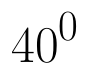(iv) 40 W         (v)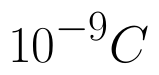(vi)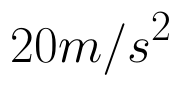(View Answer Video)

Question 4 :  Write a unit vector in the direction of the sum of vectors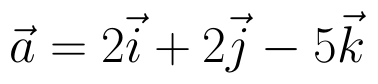and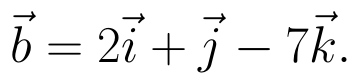(View Answer Video)

Question 5 :  Find the scalar quantities from the following:
(i) 10 kg          (ii) 2 m north-west          (iii)(iv) 40 W         (v)(vi)(View Answer Video)